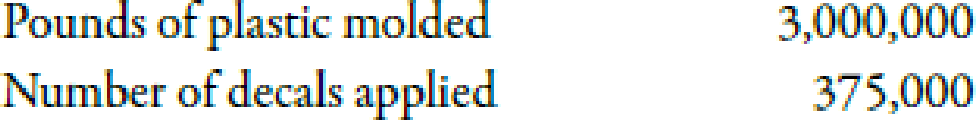Chapter 5, Problem 38E### Managerial Accounting: The Corners...

7th Edition
Maryanne M. Mowen + 2 others
ISBN: 9781337115773

#### Solutions

Chapter
Section### Managerial Accounting: The Corners...

7th Edition
Maryanne M. Mowen + 2 others
ISBN: 9781337115773
Textbook Problem
28 views

# Activity RatesPatten Company uses activity-based costing (ABC). Patten manufactures toy cars using two activities: plastic injection molding and decal application. Patten’s 20X1 total budgeted overhead costs for these two activities are $675,000 (80% for injection molding and 20% for decal application). Molding overhead costs are driven by the number of pounds of plastic that are molded together. Decal application overhead costs are driven by the number of decals applied to toys. The budgeted activity data for 20X1 are as follows:Required: 1. Calculate the activity rate for the plastic injection molding activity (round to two decimal places). 2. Calculate the activity rate for the decal application activity (round to two decimal places). 1. To determine Compute the activity rate for plastic injection molding activity. Explanation Activity Based Costing (ABC): Activity based costing is an apportionment of costs that first considers the activity drivers that helps in the allocation of costs to various activities and then allocates costs to different cost objects by using the drivers. Use the following formula to calculate activity rate for molding: Activity rate for molding=Molding activity costsPounds of plastic molded Substitute$540,000 for molding activity costs and 3,000,000 for pounds of plastic model.

Activity rate for molding=\$540,000

2.

To determine

Compute the activity rate for decal application activity.

### Still sussing out bartleby?

Check out a sample textbook solution.

See a sample solution

#### The Solution to Your Study Problems

Bartleby provides explanations to thousands of textbook problems written by our experts, many with advanced degrees!

Get Started